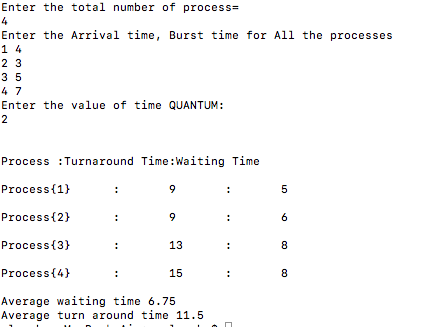# Implementation of Round Robin CPU Scheduling algorithm using C++

In this article, we are going to implement of Round Robin CPU Scheduling Algorithm (which is a preemptive version of FCFS algorithm) using C++ program.
Submitted by Aleesha Ali, on February 06, 2018

This algorithm is the preemptive version of FCFS algorithm.

Preemptive: If a process of higher priority comes then first CPU will be assign to the Process with higher priority first.

Scheduling criteria tells us that any algorithm is how much efficient, the main criteria of scheduling are given below:

• Arrival time
• Turnaround time
• Waiting time
• Burst time
• Quantum time

* Ready Queue is a queue where all the processes wait to get CPU for its execution.

Arrival time: The time at which the process enters into ready queue.

Turnaround time: The interval between the times of submission of a process to the time of completion.

Waiting time: The total amount of the time a process spends in ready queue.

Burst time: The time needed by CPU to complete its execution.

Quantum time: The amount of the time a CPU is assign to be executed is known as the quantum time independent of the actual burst time, a process will get scheduled in quantum parts values or we can say in quantum chunks.

## Round Robin Algorithm

This algorithm is known as preemptive version of FCFS as discussed earlier, it executes the process on the basis of first come first serve, and the only difference here is it works on the principle of quantum time.

## C++ Program for the Round Robin Scheduling

```//C++ Program to implement Round Robin
//Scheduling CPU Algorithm

#include <iostream>
#include <vector>

/*at = Arrival time,
bt = Burst time,
time_quantum= Quantum time
tat = Turn around time,
wt = Waiting time*/

using namespace std;

int main(){
int i,n,time,remain,temps=0,time_quantum;

int wt=0,tat=0;

cout<<"Enter the total number of process="<<endl;
cin>>n;

remain=n;
// assigning the number of process to remain variable

vector<int>at(n);
vector<int>bt(n);
vector<int>rt(n);
//dynamic array declaration using vector method of (STL)
//STL standard template library of C++

cout<<"Enter the Arrival time, Burst time for All the processes"<<endl;
for(i=0;i<n;i++)
{
cin>>at[i];
cin>>bt[i];
rt[i]=bt[i];
}

cout<<"Enter the value of time QUANTUM:"<<endl;
cin>>time_quantum;

cout<<"\n\nProcess\t:Turnaround Time:Waiting Time\n\n";
for(time=0,i=0;remain!=0;)
{
if(rt[i]<=time_quantum && rt[i]>0)
{
time += rt[i];
rt[i]=0;
temps=1;
}

else if(rt[i]>0)
{
rt[i] -= time_quantum;
//Subtraction using shorthand operators
time += time_quantum;
}

if(rt[i]==0 && temps==1)
{
remain--;
//Desplaying the result of wating, turn around time:
printf("Process{%d}\t:\t%d\t:\t%d\n",i+1,time-at[i],time-at[i]-bt[i]);
cout<<endl;

wt += time-at[i]-bt[i];
tat += time-at[i];
temps=0;
}

if(i == n-1)
i=0;
else if(at[i+1] <= time)
i++;
else
i=0;
}

cout<<"Average waiting time "<<wt*1.0/n<<endl;
cout<<"Average turn around time "<<tat*1.0/n<<endl;;

return 0;
}
```

Output